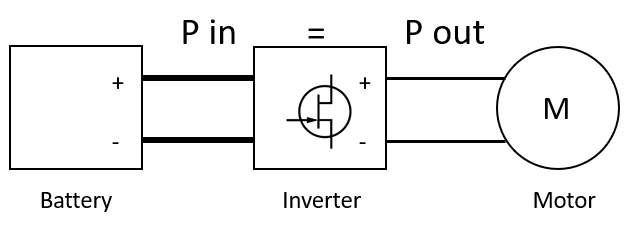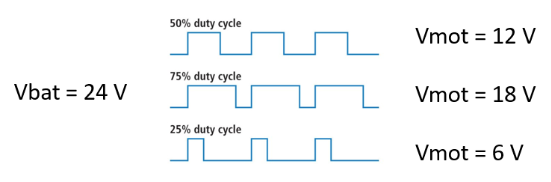One observation that usually creates questions, is the fact that during mtor's operation, the motor current can be found to be higher than the battery current. This might seem in the beginning strange, but it  can be easily explained by conceiving the controller as a system with minimum power losses, where the output power should be almost equal to the input power:This can be stated with the below equation:

Pin = Pout

Let's go now and claculate the input power (Pin)

At the input stage, the electrical power is equal to the Battery voltage multiplied by the battery current:

Pin = Battery amps * Battery voltage

The battery current is DC with a small current ripple because of the controller's input capacitors

At the output stage, the electrical power is equal to the motor voltage multiplied with the motor current

Pout = motor voltage * Motor amps

The voltage provided to the motor is the voltage obtained by the battery, modulated by the output MOSFETs using PWM. So the motor voltage is equal to the battery voltage multiplied with the PWM duty cycle.Thus we can reform the Pout equation as follows:

Pout = Battery voltage * PWM duty * Motor amps

Since Pout = Pin, we can write and develope the following equation:

Pin = Pout =>

Battery amps * Battery voltage = Battery voltage * PWM duty * Motor amps =>

Battery amps = Motor amps * PWM duty

This equation shows the following:

• At low speed, where the PWM duty required is small, the battery current will be lower than the motor current

e.g. If the motor pulls 10 A at 20% duty, the battery current will be 10 * 0.2 = 2 A

•   At high speed, where the PWM duty is high, the battery current will be close to the motor current

e.g. If the motor pulls 2 A at 95% duty, the battery current will be 2 * 0.95 = 1.9 A

By increasing the PWM duty cycle, the battery current will approach the motor current and at 100% the two currents will be alsmos identical.